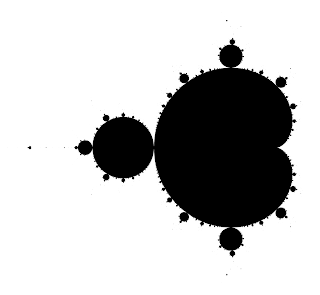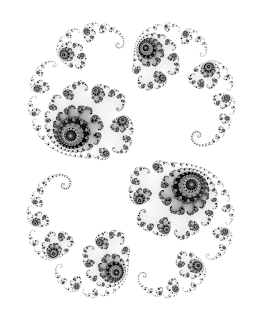## Saturday, March 2, 2013

I wrote a program (years ago) in Haskell that generated the image above of the Mandelbrot set. I really like Haskell, but I haven't spent enough time with it and never really wrapped my head around monads. I'd like to dive back into it when I get the time.

Anyway, the program I used to generate the fractal is below. I'm not a Haskell guru, so if the code is an abomination and you know how to improve it, please share in the comments! It would be cool to improve my Haskell skills. I wrote this years ago:

```module Main where

import Data.Complex
import Data.Char
import qualified Data.ByteString.Char8 as C -- For file output

-- Constants
maxIter :: Int -- Max iterations
maxIter = 750

width  :: Int -- Image width
height :: Int -- Image height

width  = 400
height = 400

-- Note: aspect ratio of (minX, minY), (maxX, maxY) must
-- match aspect ratio of (width, height)
minX :: Double -- Min x-coordinate of graph
maxX :: Double -- Max x-coordinate of graph
minY :: Double -- Min y-coordinate of graph
maxY :: Double -- Max y-coordinate of graph

-- For the zoomed in part of the Mandelbrot:
--minX = -0.826341244461360116
--maxX = -0.8026423086165848822

--minY = -0.2167936114403439588
--maxY = -0.193094675595568725

--For a full view of the mandelbrot
minX = -2.5
maxX = 1.5

minY = -2
maxY = 2

-- The actual fractal part
-- It basically works on a matrix, which we will call M, that represents a grid of
-- points on the graph. Essentially, M[i, j] is (xList[j], yList[i])
xList :: [Double]
yList :: [Double]

xList = [minX, (minX + ((maxX - minX) / (fromIntegral width  - 1)))..maxX]
yList = reverse [minY,(minY + ((maxY - minY) / (fromIntegral height - 1)))..maxY]

row :: Double -> C.ByteString -- A row of image bytes for a given y-coordinate
row y = C.pack [frac (x :+ y) (0 :+ 0) 0 | x <- xList] -- For Mandelbrot set
--row y = C.pack [frac ((-0.1) :+ (0.8)) (x :+ y) 0 | x <- xList] -- For Julia set

etaFraction :: Complex Double -> Double
etaFraction z = (log (log (magnitude z))) / log 2

smoothEta :: Int -> Complex Double -> Double -- Smooth escape time algorithm value
smoothEta iter z = (fromIntegral iter - etaFraction z) / fromIntegral maxIter

color :: Int -> Complex Double -> Double -- Gets the color for the point, in range [0, 1]
color iter z = 1 - smoothEta iter z -- Smooth escape time algorithm (and invert)
--color iter z = fromIntegral iter / fromIntegral maxIter

interpolate :: Double -> Char -- Adds an interpolation curve for interpolating color
interpolate v = chr (truncate ((v ^ 12) * 255)) -- Polynomial curve
--interpolate v = chr (truncate(v * 255)) -- Linear

frac :: Complex Double -> Complex Double -> Int -> Char -- The actual fractal algorithm!
frac c z iter
| iter >= maxIter = chr 255 -- never escaped, return color value of 255
| otherwise = let z' = z * z + c
in if ((realPart z') * (realPart z') + (imagPart z') * (imagPart z')) > 4
then interpolate (color iter z')
else frac c z' (iter + 1)

-- The file output
pgmHeader = C.pack ("P5\n" ++ (show width) ++ " " ++ (show height) ++ "\n255\n")
main = C.writeFile "fractal.pgm" (C.append pgmHeader (C.concat [(row y) | y <- yList]))
```

And some pictures from this program (each image has had some constants tweaked):The Mandelbrot set in pure black and white; only pixels considered "inside" the set are black.A Julia set based on (0.285, -0.01i). I actually edited the brightness/contrast in post-processing on this one to really bring out the spirals.A zoomed in portion of the Mandelbrot set. I edited this one's brightness/contrast settings in post processing to bring out some of the little details.